Next: Measurement of vertical displacements Up: Results Previous: Measurement of

### Measurement of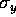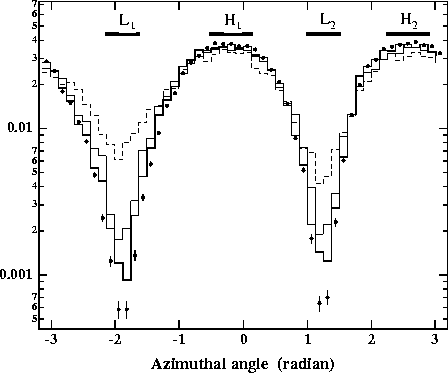Figure 7:   Azimuthal angular distributions measured for 6< r < 7cm at z=+1m for R=85.5(solid circles), 42.8(solid line), 28.5(dotted one) and 8.55(dashed one) corresponding to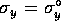, 2, 3and 10, respectively. The distributions are normalized by the total number of particles. The angular regions of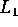(left),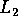(right),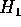(down) and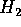(up) are also shown.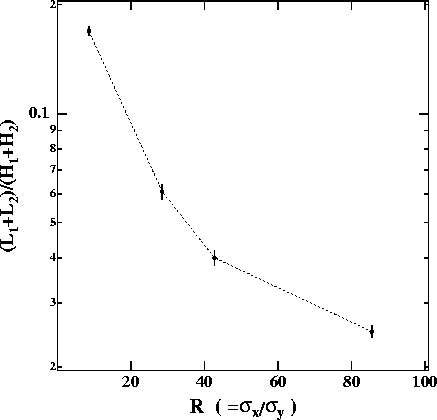Figure 8:   Number of particles inandnormalized by that inandas a function of R. The same data are used as in the previous figure. The errors are statistical, corresponding to 160 bunch crossings, except for the lowest R, which corresponds to 800 bunch crossings.

Figure 7 shows the azimuthal angular distributions measured for 6< r < 7cm at z=+1m for various values of's, where 4 angular regions are defined for further numerical studies, i.e.(left)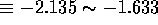,(right)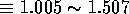,(down)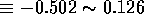and(up)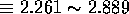. By comparing this figure with Fig.4 we can clearly see that the linear dependence on R of the yields in the depletion region is preserved through the motion in the solenoid. The effect of the horizontal crossing can still be seen in Fig.7; that is, there is more depletion on the left side() than on the right (). The numbers of particles are expected to be 2.4, 3.5, 120, and 120 per single-bunch crossing in,,and, respectively, under the nominal beam condition. The statistics will actually be much better because the beam pulse contains 72 bunches. Thus, there will be no problem in the statistical sensitivity for this measurement, although the yields decrease inversely proportional to. The ratio of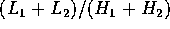is plotted as a function of R in Fig.8. Actually, this ratio depends only on R, as expected by Eq.13. Since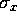is measured from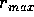, we can obtain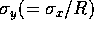by this azimuthal angular distribution. The statistical accuracy will be less than 0.1with a few pulse-train crossings if no significant background exists.Next: Measurement of vertical displacements Up: Results Previous: Measurement of

Toshiaki Tauchi
Sat Dec 21 00:34:16 JST 1996